Science Courses / Course / Chapter

# Adding & Subtracting Negative Fractions

Lesson Transcript
Instructor: Mia Primas

Mia has taught math and science and has a Master's Degree in Secondary Teaching.

Negative fractions are representing partial numbers that are less than zero, and may be easier to work with in the context of time and money. See how to add and subtract negative fractions through examples of time changes and money debt. Updated: 11/20/2021

## Working with Negative Numbers

So, you know how to add, you know how to subtract, you know what fractions are and how to add them. So, what's the next step? Well you may remember that not all numbers are positive numbers. If you think of a number line, the line of numbers goes out from zero in both directions. To the right, we have all the positive numbers, and to the left of zero, are all of our negative numbers. In between the whole numbers, there are even negative parts of numbers, which may be written as fractions.

In this lesson, we'll look at how to add and how to subtract negative fractions. When working with negative numbers, or numbers that are less than zero, it's often helpful to think of them in terms of money or time. Having negative values for money or time means that we owe that amount.

First let's look at an example that will show you how to subtract fractions as they apply to time.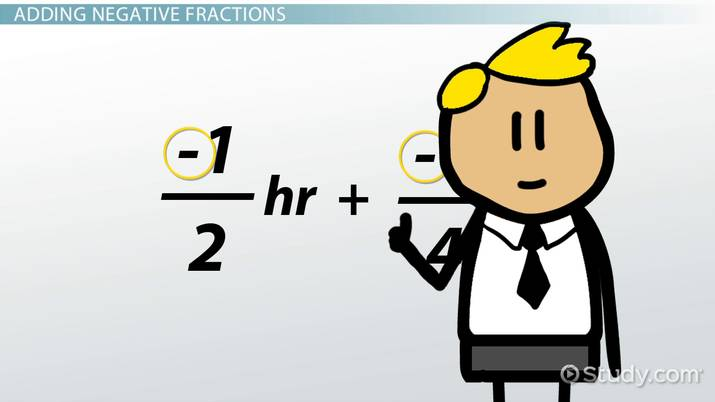An error occurred trying to load this video.

Try refreshing the page, or contact customer support.

Coming up next: Common Denominator: Finding & Fractions

### You're on a roll. Keep up the good work!

Replay
Your next lesson will play in 10 seconds
• 0:00 Working with Negative Numbers
• 2:43 Subtracting a Negative…
• 4:31 Lesson Summary
Save Save

Want to watch this again later?

Timeline
Autoplay
Autoplay
Speed Speed

My son gets a behavior report every day from school. Based on how well he did in school, he gets rewarded with free time to play with his friends or to watch TV. If his report is poor, he owes me time by doing extra chores around the house.

Last week was an especially challenging week for him. On Monday, he had 3 negative comments from his teacher, so he owed me 30 minutes of chores. Tuesday was a little better, but he still owed 15 more minutes of chores. Mathematically, we would represent his total time owed as: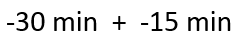We would add the total minutes and the result would be -45 minutes, meaning that he owes me a total of 45 minutes of chores. But this lesson is about fractions, so let's re-write our equation using hours as our units. Our equation now becomes: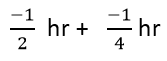Notice that I placed the negative sign in the numerator. With negative fractions, the negative sign can be placed in the front of the fraction, in the numerator, or in the denominator. I find it most helpful to keep it in the numerator when doing calculations.

Let's continue with our calculation. It is important to remember that when adding or subtracting fractions, they must have a common denominator, which means that the number on the bottom of both of the fractions have to be the same. We can change the first fraction to -2/4 so that both fractions have a denominator of 4.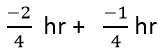Next, we add the numerators and keep the denominator the same.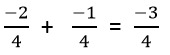Our total is -3/4 hours. So, for Monday and Tuesday, my son owed me 3/4 of an hour of chores. This is equal to the -45 minutes that we calculated earlier.

Now let's take a look at how to subtract negative fractions.

To unlock this lesson you must be a Study.com Member.

### Register to view this lesson

Are you a student or a teacher?

Back

### Resources created by teachers for teachers

Over 30,000 video lessons & teaching resources‐all in one place.Video lessonsQuizzes & WorksheetsClassroom IntegrationLesson Plans

I would definitely recommend Study.com to my colleagues. It’s like a teacher waved a magic wand and did the work for me. I feel like it’s a lifeline.

Jennifer B.
TeacherCreate an account to start this course today
Used by over 30 million students worldwide